# Hands-on Digital transmission with your soundcard¶

## 04 - Passband signal transmission¶

In the previous notebook we experienced that the soundcard does have significant attenuation in the lower frequency region. Hence, baseband transmission with RC filtered signals was not possible due to the strong distortion of the signal. As a result, we could not get a clear eye diagram of the received signal.

In this notebook, we will move the signal from baseband to passband, i.e. upconvert the signal to some carrier frequency. Note, that we still use real-valued signals for simplicity.

To further simplify the system, we use double-side band AM with large-carrier modulation (DSB-AM LC). In this system, we perform amplitude modulation of the carrier signal, given by

$$s(t) = (1+g\cdot x(t))\cos(2\pi f_c t)$$

where $s(t)$ is the transmitted signal, $x(t)$ is the baseband signal, $g$ is the modulation index and $f_c$ is the carrier frequency. With this modulation, we need to ensure that $g\cdot max(|x(t)|)<1$ such that the carrier is always present in the signal.

Let's start this notebook with the standard imports:

In :
import matplotlib
import matplotlib.pyplot as plt
%matplotlib notebook

import numpy as np
import sys; sys.path.append('..')

In :
try:
except ModuleNotFoundError:
print ("Did not find tikzmagic. You will not be able to compile the tex code!")

In :
%matplotlib notebook

In :
# Source code has been redacted in online version
# Omitting 4 lines of source code


Moreover, let's get our standard baseband transmitter from the previous notebook.

In :
# Source code has been redacted in online version
# Omitting 19 lines of source code


Let's create an upconverted version of the signal and send it through some channel. Therefore, we use the Upconversion block which is provided by the accompanying library. As the receiver, we use a signal recorder, which records the signal for a given amount of time and automatically stops the transmission afterwards. Subsequently, we have a look at the transmitted signal:

In :
from audioComms.components import Upconversion, Recorder

def recordPassbandSignal(channelFunc):
samplerate = 44100
Fc = 3000 # carrier frequency
env = Environment(samplerate=samplerate)

Ts = 1/(441)  # symbol duration
t = np.arange(-4*Ts, 4*Ts, 1/samplerate)

transmitter = TransmitSignal(env, get_filter('rc', Ts, rolloff=0.5)(t), Ts)
upconversion = Upconversion(env, Fc=Fc)
channel = channelFunc(env)
recorderBB = Recorder(env, duration=1)  # record signal for one second
recorderUP = Recorder(env, duration=1)

transmitter.transmitsTo(upconversion)
transmitter.transmitsTo(recorderBB)

upconversion.transmitsTo(channel)
upconversion.transmitsTo(recorderUP)

env.run()

plt.figure(figsize=(8,3))
plt.plot(0.2+0.1*recorderBB._samples, lw=4, color='r', label='Envelope')
plt.plot(recorderBB._samples, label='Baseband signal')
plt.plot(recorderUP._samples, label='Passband signal')
plt.legend()

plt.xlim((10000, 12000))
recordPassbandSignal(lambda env: SimulatedChannel(environment=env, channelEffect=IdentityChannelEffect(gain=0.2)))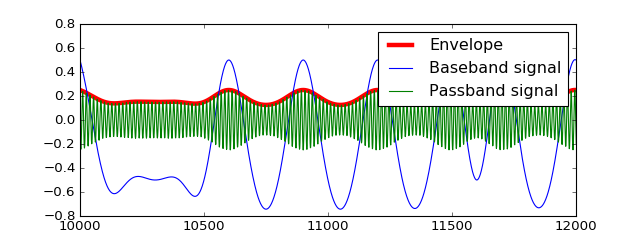Apparently, the baseband signal is reflected as the envelope of the transmit passband signal. In addition, the carrier is clearly visible all the time.

Now, let's actually transmit the signal over the channel and perform downconversion at the receiver side. For downconversion, we use the Downconversion block, which is supplied by the accompanying library. In addition to performing the downconversion, this block removes the carrier from the signal and subtracts the mean from the baseband signal, yielding the original signal back. At the receiver side, we plot the eye diagram and receive spectrum. Pictorially, we have the following blocks in the chain:

In :
# Source code has been redacted in online version
# Omitting 25 lines of source codeIn :
from audioComms.components import Downconversion
def runTransmission(Fc, channelFunc):
samplerate = 44100
env = Environment(samplerate=samplerate)

Ts = 1/(441)  # symbol duration
t = np.arange(-4*Ts, Ts, 1/samplerate)
transmitter = TransmitSignal(env, get_filter('rc', Ts, rolloff=0.5)(t), Ts)
upconversion = Upconversion(env, Fc=Fc)

channel = channelFunc(env)

downconversion = Downconversion(env, Fc=Fc, B=4/Ts, removeCarrier=True)
plotSpec = PlotSpectrum(env, windowDuration=0.1, logScale=True, figsize=(8,3))
plotEye = PlotEyeDiagram(env, Ts, figsize=(8,3))

transmitter.transmitsTo(upconversion)
upconversion.transmitsTo(channel)
channel.transmitsTo(plotSpec)
channel.transmitsTo(downconversion)
downconversion.transmitsTo(plotEye)

env.run()


First, let's run the bandpass transmission over the simulated highpass channel:

In :
runTransmission(18000, lambda env: SimulatedChannel(env, channelEffect=HighpassChannelEffect(gain=0.3)))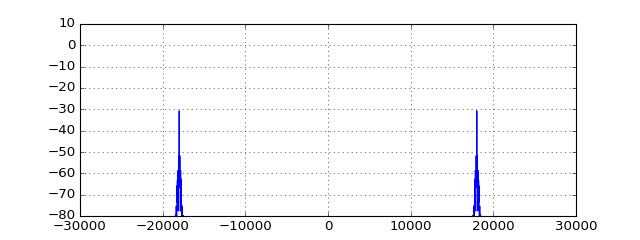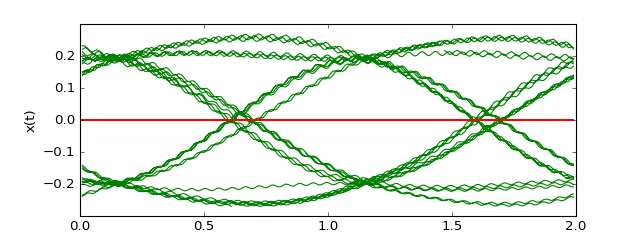Stop received from external


Nice! We get a correct and smooth eye diagram. Now, let's go ahead and run it in the real audio channel:

In :
runTransmission(18000, lambda env: AudioChannel(env))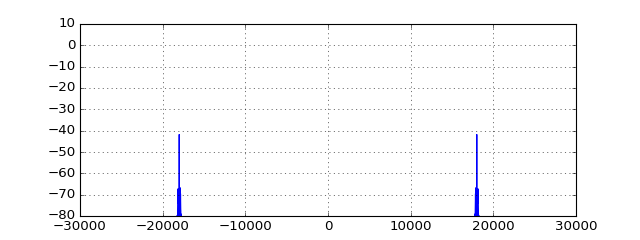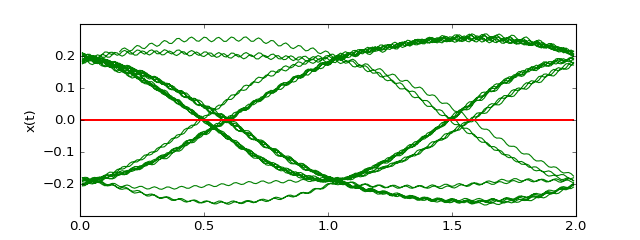Stop received from external


Nice! Also the audio channel gives us a nice eye diagram! In addition, the 18kHz carrier frequency are well above what you can hear. However, note that your dog or cat might become quite annoyed. Try setting the carrier frequency to a lower frequency, e.g. 5kHz:

In :
runTransmission(5000, lambda env: AudioChannel(env))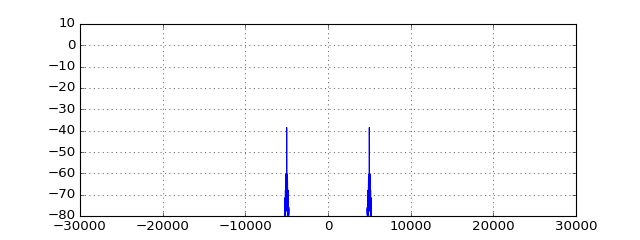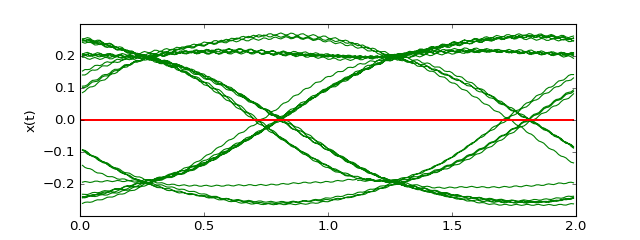Stop received from external


The eye diagram is still nicely visible, but the tone quickly becomes annoying. Better switch back to 18kHz.

### Summary and Outlook¶

Now that we have a working eye diagram, we are ready to sample the received signal and detect the transmitted bits, right? Well, not yet.

Have you observed that the eye opening always appears at a different position in the eye diagram? Did you observe, that the opening might even move during the transmission over the audio channel? In order to sample the eye diagram, one has to optimally sample the eye pattern at the time of largest opening. However, this information is not yet available. We will tackle this timing recovery problem in the next notebook, using the Gardner Algorithm.